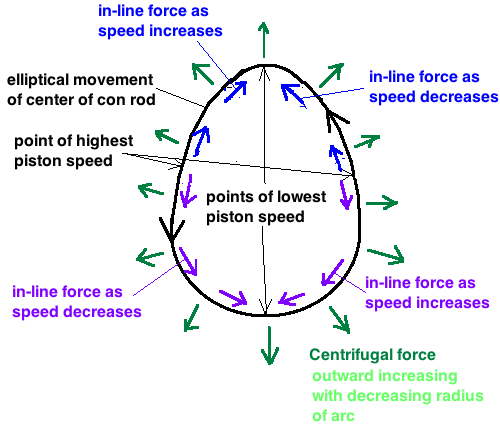General Physics General Physics Help ForumJun 6th 2018, 11:24 PM #1 Junior Member   Join Date: Jun 2018 Location: Paraguay Posts: 4 inertia formula for con rod I am trying to calculate all the forces (in bf) that the connecting rod adds to the engine which influences vibration. I am dividing the rod in between the two ends into four sections and calculating for the middle point of each as if that point had all that sections weight. I am calculating for every 15 degrees of crank rotation.Heres the formulas I have but Im not sure about the in-line force formula: centrifugal force in lbf = (kg x (meters/sec velocity)^2 / arc radius in meters) x .225 in-line force in lbf = (kg x (meters/sec beginning velocity^2) / 2) x .74 I am smudging a bit by ignoring the curvature of movement for calculating the in-line force but I dont care if its 10% off. I think inertia force is the same as what Im calling in-line force because to stop a moving object you have to exert a force equal to its inertia. Correct me if Im wrong.Tags con, formula, inertia, rodThread ToolsShow Printable VersionEmail this Page Display ModesLinear ModeSwitch to Hybrid ModeSwitch to Threaded ModeSimilar Physics Forum Discussions Thread Thread Starter Forum Replies Last Post Toan Special and General Relativity 27 Feb 26th 2018 06:35 PM ling233 Advanced Mechanics 0 Jan 20th 2016 04:11 PM aman Light and Optics 1 Jun 1st 2009 10:32 PM jonbrutal Advanced Mechanics 3 Apr 2nd 2009 05:04 AM kdaaa Advanced Mechanics 1 Mar 11th 2009 12:48 AM